### Joule Cycle

Joule cycle consists of two constant pressure and two reversible adiabatic or isentropic processes as shown on p - v and T-s diagram in Fig. 5.10 (a) and (b) respectively.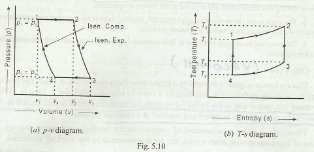The efficiency of Joule cycle is given by equation (i):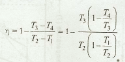We know that for reversible adiabatic or isentropic expansion 2 – 3,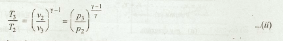and for reversible adiabatic or isentropic compression 4 – 1,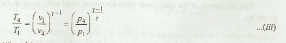From equations (ii) and (iii),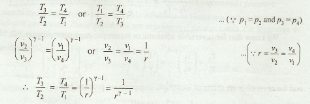Now equation (i) may be written as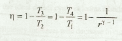Notes:

1. The efficiency of the Joule's cycle is lower than Carnot efficiency. The reason is that all the heat is not taken in at the highest temperature and rejected at the lowest temperature.

2. The cycle is not thermodynamically reversible, because there is no re-generator to provide a constant temperature during heating and cooling at constant pressure.

3. The reversed Joule cycle is known as Bell-coleman cycle, and is applied to refrigerators, where air is used as a refrigerant.